Courses

# NCERT Solutions (Ex - 9.1,9.2,9.3) - Algebraic Expressions, Class 9, Maths Class 8 Notes | EduRev

Created by: Indu Gupta

## Class 8 : NCERT Solutions (Ex - 9.1,9.2,9.3) - Algebraic Expressions, Class 9, Maths Class 8 Notes | EduRev

The document NCERT Solutions (Ex - 9.1,9.2,9.3) - Algebraic Expressions, Class 9, Maths Class 8 Notes | EduRev is a part of Class 8 category.
All you need of Class 8 at this link: Class 8

Exercise 9.1

Question 1: Identify the terms, their coefficients for each of the following expressions:

[i] 5xyz2 - 3zy

[ii] 1 +x+ x2

(iii) 4x2y2 - 4x2y2z2 + z2

[iv] 3 - pq + qr — rp

(v) x/2 + y/2 - xy

[vi] 0.3a - 0.6ab + 0 5b

(i)

Terms: 5xyz2 - 3zy

Coefficient in x is 5 and in -3zy is -3

(ii)

Terms:  1 +x+ x2

Coefficient of x and coefficient of x2 is 1.

(iii)

Terms: 4x2y2 - 4x2y2z2 + z2

Coefficient in 4x2y2 is 4, coefficient of -4x2y2z2 is -4 and coefficient of zis 1.

[iv]

Terms: 3 - pq + qr — rp

Coefficient of -pq is -1, coefficient of qr is 1 and coefficient of -rp is -1.

[v]

Terms : x/2 , y/2 , -xy

Coefficient of x/2 is 1/2, coefficient of y/2 is 1/2 and coefficient of -xy is -1.

[vi]

Terms: 0.3a,-0.6ab and 0.56

Coefficient of 0.3a is 0.3, coefficient of -0.6ab is -0.6 and coefficient of 0.5b is 0.5

Question 2:

Classify the following polynomials as monomials, binomials, trinomials. Which polynomials do not fit in any of these three categories:

1. x + y
2. 1000,
3. x + x2 + x3 + x4
4. 7 +y + 5x,
5. 2y-3y2
6. 2y-3y2 + 4y3
7. 5x-4y+3xy,
8. 4z-15z2
9.  ab + bc + cd + da,
10. pqr
11. p2q + pq2
12. 2p + 2q

1. Since x + y contains two terms. Therefore it is binomial.
2. Since 1000 contains one terms. Therefore it is monomial.
3. Since x + x2 + x2 +x4 contains four terms. Therefore it is a polynomial and it does not fit in above three categories.
4. Since 7 y + 5x contains three terms. Therefore it is trinomial.
5. Since 2y-3y2 contains two terms. Therefore it is binomial.
6. Since 2y-3y2 + 4y3  contains three terms. Therefore it is trinomial.
7. Since 5x-4y 3xy contains three terms. Therefore it is trinomial.
8. Since 4x -15y2 contains two terms. Therefore it is binomial.
9. Since ab+hc+cd da contains four terms. Therefore it is a polynomial and it does not fit in above three categories.
10. Since pqr contains one terms. Therefore it is monomial.
11. Since p2q + pq2 contains two terms. Therefore it is binomial.
12. Since 2p + 2q contains two terms. Therefore it is binomial

Question 3: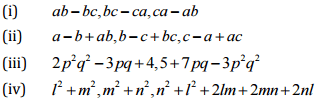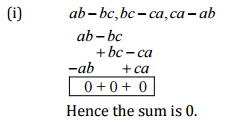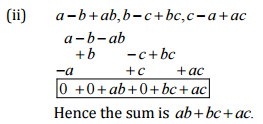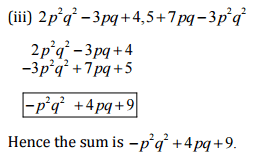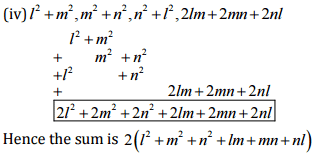Question 4:

(a) Subtract 4a-7zb + 3b + 12 from 12a-9ab+5b-3

(b) Subtract 3xy + 5yz - 7zx from 5xy - 2yz - 2zx + 10 xyz

(c) Subtract 4p2q - 3pq + 5pq2 - 8p + 7q - 10 from 18- 3p-11q+5pq-2qp2 + 5 p2q

(a)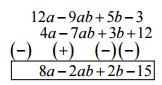(b)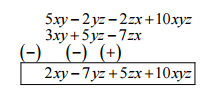(c)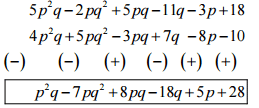Exercise 9.2

Question 1:

Find the product of the following pairs of monomials:

(i) 4, 7p

(ii) -4p, 7p

(iii) - 4p, 7pq

(iv) 4p3, - 3p

(v) 4p, 0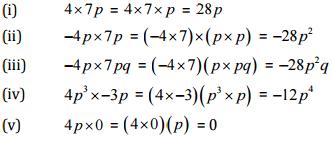Question 2:

Find the areas of rectangles with the following pairs of monomials as their lengths and breadths respectively:
(p,q); (10m,5n); (20x2,5y2); (4x,3x2); (3mn,4np)

[i] Area of rectangle = length x breadth

= pxq = pq sq. units

[ii] Area of rectangle = length x breadth

= 10m  x 5n = (10x5)(mxn) = 50 mn sq. units

[iii] Area of rectangle = length x breadth

= 20x2 x 5y2 = (20 x 5 ) (x2 x y2) = 100x2y2 sq. units

(iv) Area of rectangle = length x breadth

= 4x x 3x2 = (4x3)(x x x2 ) sq. units

(v) Area of rectangle = length x breadth

= 3mn x 4np = (3x4)(mn x np) = 12mn2p sq. units

Question 3:

Complete the table of products:

 First monomial  →Second monomial 2x —5y 3x2 -4 xy 7x2y -9 x2y2 2x 4x2 ..... ..... ..... ..... ..... -5y ..... ..... -15x2y ..... ..... ..... 3x2 ..... ..... ..... ..... ..... ..... -4 xy ..... ..... ..... ..... ..... ..... 7x2y ..... ..... ..... ..... ..... ..... -9x2y2 ..... ..... ..... ..... ..... .....

 First monomial  →Second monomial 2x —5y 3x2 -4 xy 7x2y -9 x2y2 2x 4x2 -10xy 6x3 -8x2y 14x3y -18x3y2 -5y -10xy 25 y2 -15x2y 20 xy2 -35x2y2 -45 x2y3 3x2 6x3 -15x2y 9x4 -12x3y 21 x4y -27 x4y2 -4 xy 8x2y 20 xy2 -12x3y 16x2y2 -28 x3y2 36 x3y3 7x2y 14x3y -35 x2y2 21 x4y -28x3y2 49 x4y2 -63 x4y3 -9x2y2 -18x3y2 45x2y3 -27 x4y2 36 x3y3 -63x4y3 81 x4y4

Question 4:

Obtain the volume of rectangular boxes with the following length, breadth and height respectively:

(i) 5a, 3a27a4

(ii) 2p,4q18r

(iii) xy, 2x2y, 2xy2

(iv) a, 2b, 3c

(i) Volume of rectangular box = length x breadth x height

= 5a x 3 a2 x 7a4 = ( 5x3x7)(a x a2 x a4)

= I05a7 cubic units

(ii) Volume of rectangular box = length x breadth x height

= 2p x 4 q x 8r = ( 2x4x8)(pxqxr)

= 64 pqr cubic units

(iii) Volume of rectangular box = length x breadth x height

= xy, 2x2y, 2xy2  = (1 x 2 x 2 )(x x x2 x yx yx y2)

= 4x4 y2 cubic units

(iv)

Volume of rectangular box = length x breadth x height

= a x 2b x 3c = (1x2x3) (axbxc) 6abc cubic units

Question 5:

Obtain the product of:

(i) xy,yz,zx

(ii) a, -a2, a3

(iii) 2, 4y, 8y2, 16y3

(iv) a,2b,3c,6abc

(v) m, -mn,mnp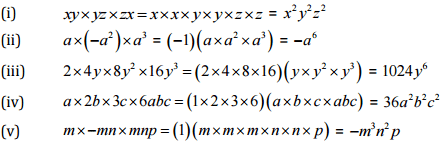Exercise 9.3

Question 1:

Carry out the multiplication of the expressions in each of the following pairs:

(i) 4p,q+r

(ii) ab, a-b

(iii) a+ b, 7a2b2

(iv) a2- 9, 4a

(v) pq+qr+rp,0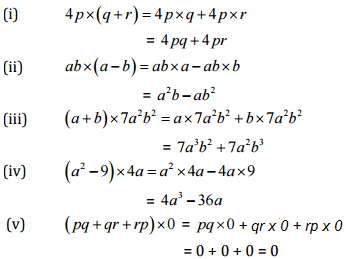Question 2:

Complete the table: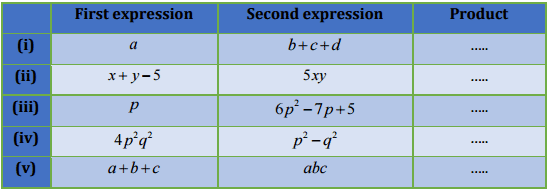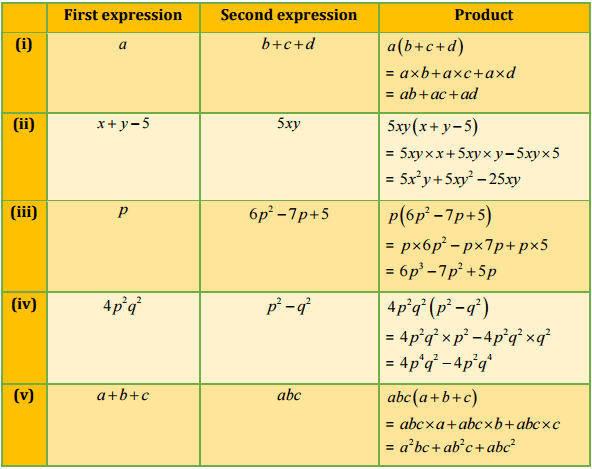Question 3:

Find the product: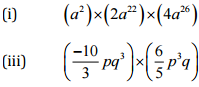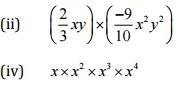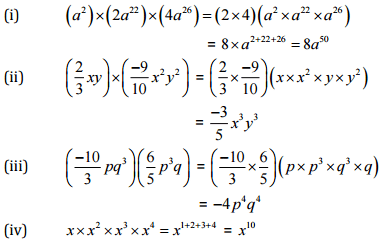Question 4:

(a) Simplify: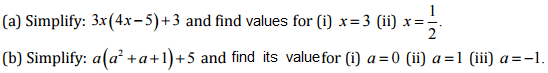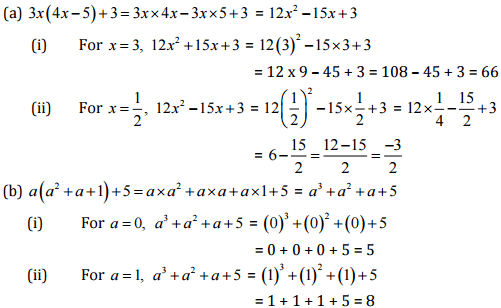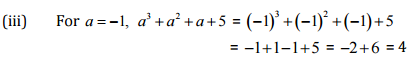Question 5: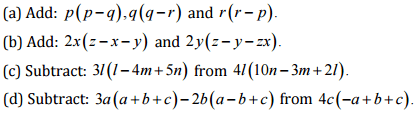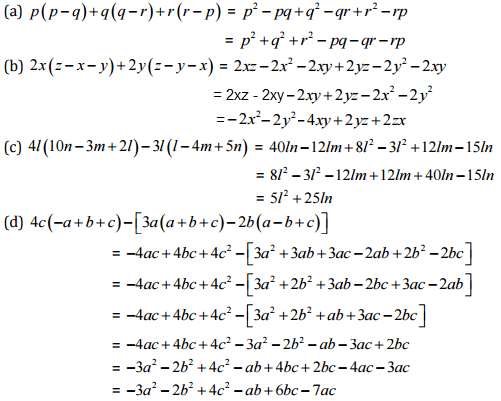,

,

,

,

,

,

,

,

,

,

,

,

,

,

,

,

,

,

,

,

,

,

,

,

,

,

,

,

,

,

,

,

,

,

,

,

,

,

;